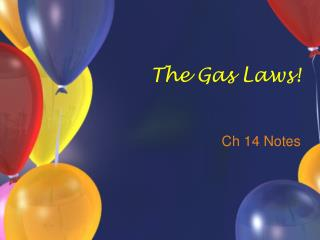DownloadDownload PresentationThe Gas Laws!

# The Gas Laws!

Download Presentation## The Gas Laws!

- - - - - - - - - - - - - - - - - - - - - - - - - - - E N D - - - - - - - - - - - - - - - - - - - - - - - - - - -
##### Presentation Transcript

1. The Gas Laws! Ch 14 Notes

2. Properties of Gases • Gases are compressible because of the space between particles. • Factors that affect gases: • Pressure • Volume • Temperature • # of moles present

3. Boyle’s Law • P1V1 = P2V2 • Inverse Relationship • T is constant • If one goes up, the other goes down • If one goes down, the other goes up

4. Lung model, marshmellows, bell jar, crush the can

5. Boyle’s Law Sample Prob • You and your crazy friend want to make funny voices using helium. You obtain a helium tank…. The volume of the gas in the tank is 2 L, but can fill over 200 L at room pressure. Room pressure is 1 atm, what was the original pressure?

6. Charles’ Law • V1/T1 = V2/T2 • Direct Relationship • P is constant • Temp in Kelvin!!! (°C + 273) • As one goes up, so does the other • As one goes down, so does the other • higher temp = more kinetic energy, more collisions, so volume increases

7. Charles’ Law Sample Prob • The temperature in the supermarket is a frosty 15 °C. When you buy your bag of chips it occupies 1 L of space. You leave the chips in your car for 1 hour and it reaches a temperature of 27 °C. what is the new volume of the bag?

8. Gay-Lussac’s Law • P1/T1 = P2/T2 • Direct Relationship • Temp in Kelvin!!! • Volume is constant • Sample: The temperature of a gas goes from 30 °C to 50 °C. The starting pressure is 760 mm Hg, what is the ending pressure?

9. Go To Absolute Zero Video Clip

10. Combined Gas Law • P1V1/T1 = P2V2/T2 • Combines Boyle’s, Charles’, and Gay-Lussac’s Laws • Sample: Your mom gives you a balloon that says “I love you!” (awww!). When she hands it to you it has a pressure of 1.5 atm and is at 295 K. The next morning it is at 290 K, has a pressure of 1.2 atm and has a volume of 1.5 L. What was the original volume of the balloon? http://www.youtube.com/watch?v=xwKpXEUds0c&NR=1

11. Molar Volume • At STP, 1 mole of any gas occupies 22.4 L • STP = 0 °C (273 K) and 1 atm • What will the volume of 5 moles of gas be at STP? • A sample of gas occupies 11.2 L at STP, how many moles is in the sample? • How many atoms are in 5 L of gas at STP?

12. Ideal Gas Law • relates P, V, T, and number of moles (n) • PV = nRT • R = 0.0821 (L*atm)/(mol*K) = “ideal gas law constant” • R = 8.3 (L*kPa)/(mol*K) • ‘ideal’ gases - particles have no volume & no attractive forces • real gases are ‘ideal’ gases under all conditions except very high pressure or very low temperature go to animation

13. Sample Problems… • You have 4 moles of helium gas at 1.5 atm and 25 L, what is the temperature? • How many moles of argon gas are present in 500 mL at 3 atm and 30 ºC?

14. Diffusion • movement from high to low concentration • caused by random collisions in which no energy is lost • rate of diffusion depends on temperature (amt of KE) and the mass of the particles air sprays salt in beaker dye in beaker…

15. Dalton’s Law of Partial Pressures • At a specific temperature and pressure, the ‘partial’ pressure of one mole of gas is the same regardless of the gas identity. • In a gas mixture, the total pressure equals the sum of the pressure of each gas. • P total = P1 + P2 + P3 + …. Pn

16. sToP & tHinK • You are asked to add chlorine to your neighbor’s swimming pool and spa while they’re out of town. In which one will the chlorine totally spread out in the liquid faster? • Use the assumptions from kinetic molecular theory to explain! • A sample of gas has a total pressure of 5 atm. It contains argon and one unknown gas. The argon contributes 3.5 atm of pressure. What is the pressure of the unknown gas? • Can we use this information to figure out the identity of the gas?!?!

17. Gas Stoichiometry • Coefficients in balanced reaction equations are mole ratios AND gas volume ratios! • Can do “vol to vol” stoichiometry when P and T are constant

18. Volume - Volume Gas Stoich (These are just like mole to mole problems - one conversion!) How many liters of NH3 will be produced if 10 liters of N2 are consumed according to the following reaction: N2 (g) + 3H2 (g) -----> 2NH3 (g)

19. Volume-Mass Gas Stoich Example N2 (g) + 3H2 (g) -----> 2NH3 (g) How many grams of hydrogen gas are required to create 20 L of ammonia gas if the above reaction occurs at STP? *do volume to volume stoich, then molar volume, then molar mass.

20. Mass-Volume Gas Stoich Example N2 (g) + 3H2 (g) -----> 2NH3 (g) How many liters of ammonia gas can be created from 50 grams of nitrogen gas at STP? *mass to mole stoich, then molar volume